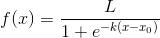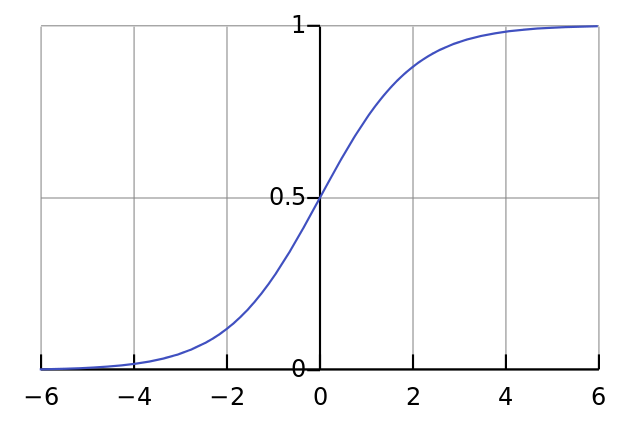# sigmoid和softmax总结

sigmoid函数（也叫逻辑斯谛函数）：
引用wiki百科的定义：

A logistic function or logistic curve is a common “S” shape (sigmoid curve).

其实逻辑斯谛函数也就是经常说的sigmoid函数，它的几何形状也就是一条sigmoid曲线。logistic曲线如下：softmax is a generalization of logistic function that “squashes”(maps) a K-dimensional vector z of arbitrary real values to a K-dimensional vector σ(z) of real values in the range (0, 1) that add up to 1.

这句话既表明了softmax函数与logistic函数的关系，也同时阐述了softmax函数的本质就是将一个K

softmax函数形式如下：